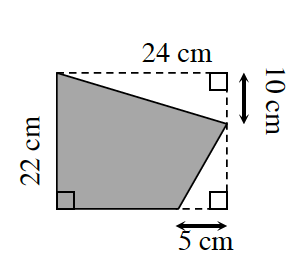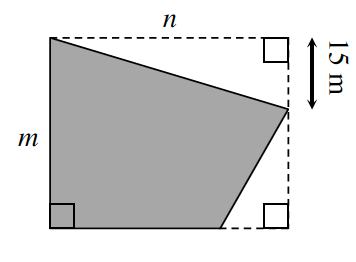### Home > INT2 > Chapter 4 > Lesson 4.2.2 > Problem4-74

4-74.

The shaded figures below are similar.1. Solve for $m$ and $n$.

Similar figures have proportional side lengths that can be written as equivalent ratios.

2. Calculate the area and perimeter of each figure.

Calculate the area of each outlined rectangle.

Then, subtract the areas of the blank spaces.

$\text{area (small)}=378 \text{ cm}^2$
$\text{perimeter (big)} = 120\text{ m}$